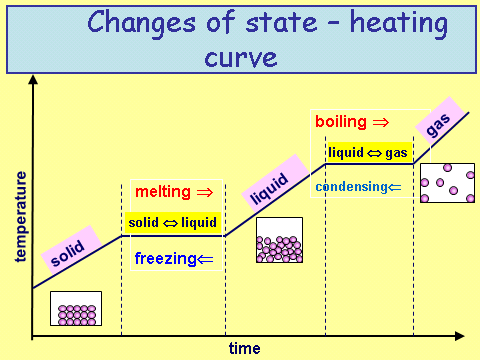# Internal Energy

## Internal Energy

Internal energy is a measure of how much energy a substance has.

It is made up of two different stores of energy: kinetic and potential.

The kinetic energy is a measure of how much the molecules/particles are moving around. This means that a gas has more energy in the kinetic store than a solid because the particles can move around much more

The potential energy is a measure of how far away the molecules/particles are from each other. The greater the difference the greater the energy in the potential store as there is a large distance in which they are be brought together. This means that a gas has more potential energy than a solid because there is a greater distance between the particles.

Internal energy = kinetic energy + potential energy

Revisiting our heat/cooling curve below we can see the following.

Whenever there is a gradient that is not zero, kinetic energy is being put into or out of the system. Whenever the gradient is zero, potential energy is being put into or out of the system.A substance is condensing, what energy store is being changed - kinetic or potential?
Potential
A substance is freezing, is the potential energy increasing or decreasing?
Decreasing